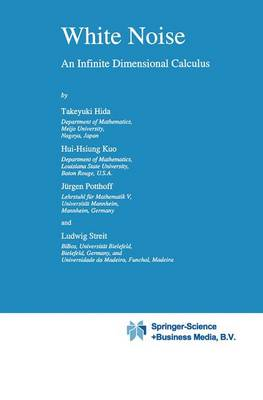Some orders may be delayed by the current Coronavirus lockdown.•# White Noise: An Infinite Dimensional Calculus - Mathematics and Its Applications 253 (Paperback)

(author), (author), (author), (author)
£109.99
Paperback 520 Pages / Published: 05/12/2010
• We can order this

Usually dispatched within 3 weeks

Many areas of applied mathematics call for an efficient calculus in infinite dimensions. This is most apparent in quantum physics and in all disciplines of science which describe natural phenomena by equations involving stochasticity. With this monograph we intend to provide a framework for analysis in infinite dimensions which is flexible enough to be applicable in many areas, and which on the other hand is intuitive and efficient. Whether or not we achieved our aim must be left to the judgment of the reader. This book treats the theory and applications of analysis and functional analysis in infinite dimensions based on white noise. By white noise we mean the generalized Gaussian process which is (informally) given by the time derivative of the Wiener process, i.e., by the velocity of Brownian mdtion. Therefore, in essence we present analysis on a Gaussian space, and applications to various areas of sClence. Calculus, analysis, and functional analysis in infinite dimensions (or dimension-free formulations of these parts of classical mathematics) have a long history. Early examples can be found in the works of Dirichlet, Euler, Hamilton, Lagrange, and Riemann on variational problems. At the beginning of this century, Frechet, Gateaux and Volterra made essential contributions to the calculus of functions over infinite dimensional spaces. The important and inspiring work of Wiener and Levy followed during the first half of this century. Moreover, the articles and books of Wiener and Levy had a view towards probability theory.

Publisher: Springer
ISBN: 9789048142606
Number of pages: 520
Weight: 813 g
Dimensions: 240 x 160 x 27 mm
Edition: 1st ed. Softcover of orig. ed. 1993